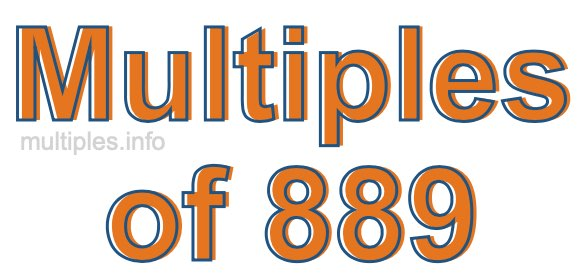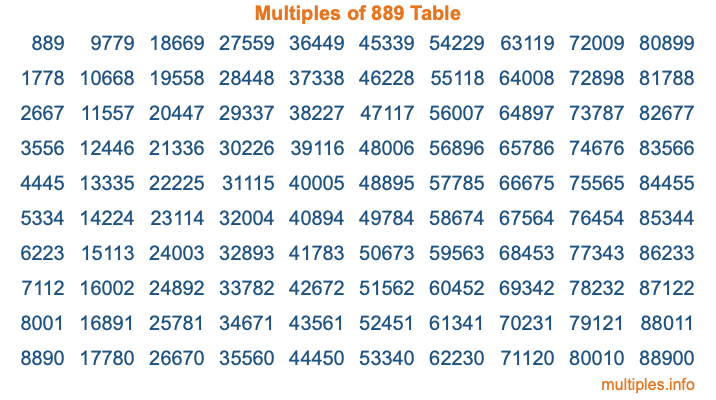Multiples of 889Welcome to the Multiples of 889 page. Here we will first teach you everything you will ever need to know about the multiples of 889, and then give you a study guide summary of everything we taught you to make sure you remember it all. Use this page to look up facts and learn information about the multiples of 889. This page will make you a multiples of eight hundred eighty-nine expert!

Definition of Multiples of 889
Multiples of 889 are all the numbers that when divided by 889 equal an integer. Each of the multiples of 889 are called a multiple. A multiple of 889 is created by multiplying 889 by an integer.

Therefore, to create a list of multiples of 889, you start with 1 multiplied by 889, then 2 multiplied by 889, then 3 multiplied by 889, and so on for as long as you want. Thus, the list of the first five multiples of 889 is 889, 1778, 2667, 3556, and 4445. To see a larger list of multiples of 889, see the printable image of Multiples of 889 further down on this page. We also have a category where you can choose any nth multiple of 889.

Multiples of 889 Checker
The Multiples of 889 Checker below checks to see if any number of your choice is a multiple of 889. In other words, it checks to see if there is any number (integer) that when multiplied by 889 will equal your number. To do that, we divide your number by 889. If the the quotient is an integer, then your number is a multiple of 889.

Is  a multiple of 889?

Least Common Multiple of 889 and ...
A Least Common Multiple (LCM) is the lowest multiple that two or more numbers have in common. This is also called the smallest common multiple or lowest common multiple and is useful to know when you are adding our subtracting fractions. Enter one or more numbers below (889 is already entered) to find the LCM.

Check out our LCM Calculator if you need more details about the Least Common Multiple or if you need the LCM for different numbers for adding and subtraction fractions.

nth Multiple of 889
As we stated above, 889 is the first multiple of 889, 1778 is the second multiple of 889, 2667 is the third multiple of 889, and so on. Enter a number below to find the nth multiple of 889.

th multiple of 889

Multiples of 889 vs Factors of 889
889 is a multiple of 889 and a factor of 889, but that is where the similarities end. All postive multiples of 889 are 889 or greater than 889. All positive factors of 889 are 889 or less than 889.

Below is the beginning list of multiples of 889 and the factors of 889 so you can compare:

Multiples of 889: 889, 1778, 2667, 3556, 4445, etc.

Factors of 889: 1, 7, 127, 889

As you can see, the multiples of 889 are all the numbers that you can divide by 889 to get a whole number. The factors of 889, on the other hand, are all the whole numbers that you can multiply by another whole number to get 889.

It's also interesting to note that if a number (x) is a factor of 889, then 889 will also be a multiple of that number (x).

Multiples of 889 vs Divisors of 889
The divisors of 889 are all the integers that 889 can be divided by evenly. Below is a list of the divisors of 889.

Divisors of 889: 1, 7, 127, 889

The interesting thing to note here is that if you take any multiple of 889 and divide it by a divisor of 889, you will see that the quotient is an integer.

Multiples of 889 Table
Below is an image of the first 100 multiples of 889 in a table. The table is in chronological order, column by column. The first column has the first ten multiples of 889, the second column has the next ten multiples of 889, and so on.The Multiples of 889 Table is also referred to as the 889 Times Table or Times Table of 889. You are welcome to print out our table for your studies.

Negative Multiples of 889
Although not often discussed or needed in math, it is worth mentioning that you can make a list of negative multiples of 889 by multiplying 889 by -1, then by -2, then by -3, and so on, to get the following list of negative multiples of 889:

-889, -1778, -2667, -3556, -4445, etc.

Multiples of 889 Summary
Below is a summary of important Multiples of 889 facts that we have discussed on this page. To retain the knowledge on this page, we recommend that you read through the summary and explain to yourself or a study partner why they hold true.

There are an infinite number of multiples of 889.

A multiple of 889 divided by 889 will equal a whole number.

889 divided by a factor of 889 equals a divisor of 889.

The nth multiple of 889 is n times 889.

The largest factor of 889 is equal to the first positive multiple of 889.

889 is a multiple of every factor of 889.

889 is a multiple of 889.

A multiple of 889 divided by a divisor of 889 equals an integer.

889 divided by a divisor of 889 equals a factor of 889.

Any integer times 889 will equal a multiple of 889.

Multiples of a Number
Here you can get the multiples of another number, all with the same attention to detail as we did for multiples of 889 on this page.

Multiples of
Multiples of 890
Did you find our page about multiples of eight hundred eighty-nine educational? Do you want more knowledge? Check out the multiples of the next number on our list!